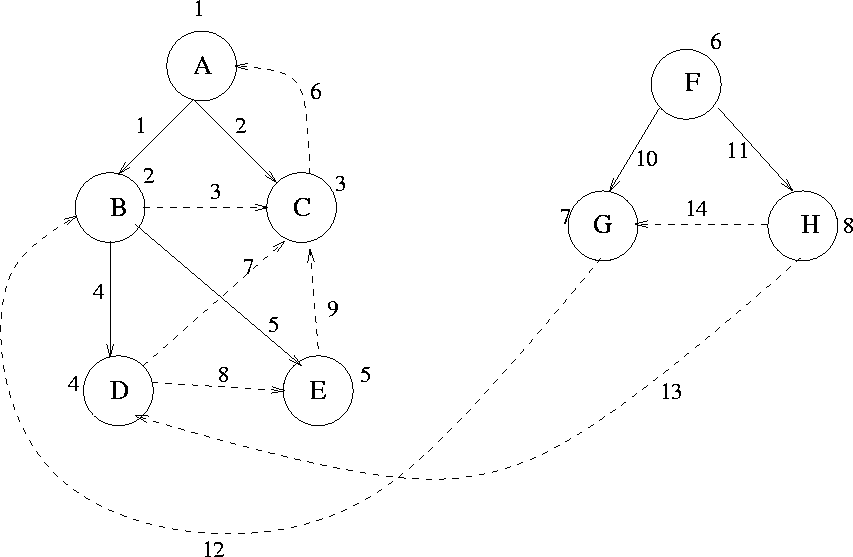Next:7.5 Directed Acyclic GraphsUp:7.4 Depth First Search and Breadth First SearchPrevious:7.4 Depth First Search and Breadth First Search

• Level by level traversal
• A queue data structure is used

•• The complexity of both DFS and BFS is O(e).

void bfs (v) /* visits all vertices connected to v

vertex x, y;

vertexqueue Q;

{

mark [v] = visited ;

enqueue (v, Q);

while (Q not empty)

{ x = front (Q) ;

dequeue (Q) ;

for (each vertex y adjacent to x)

if (mark[y] = unvisited)

{

mark[y] = visited ;

enqueue(y,Q) ;

insert ((x,y), T)

}

}

}

Example: See Figure 7.11.

eEL,CSA_Dept,IISc,Bangalore